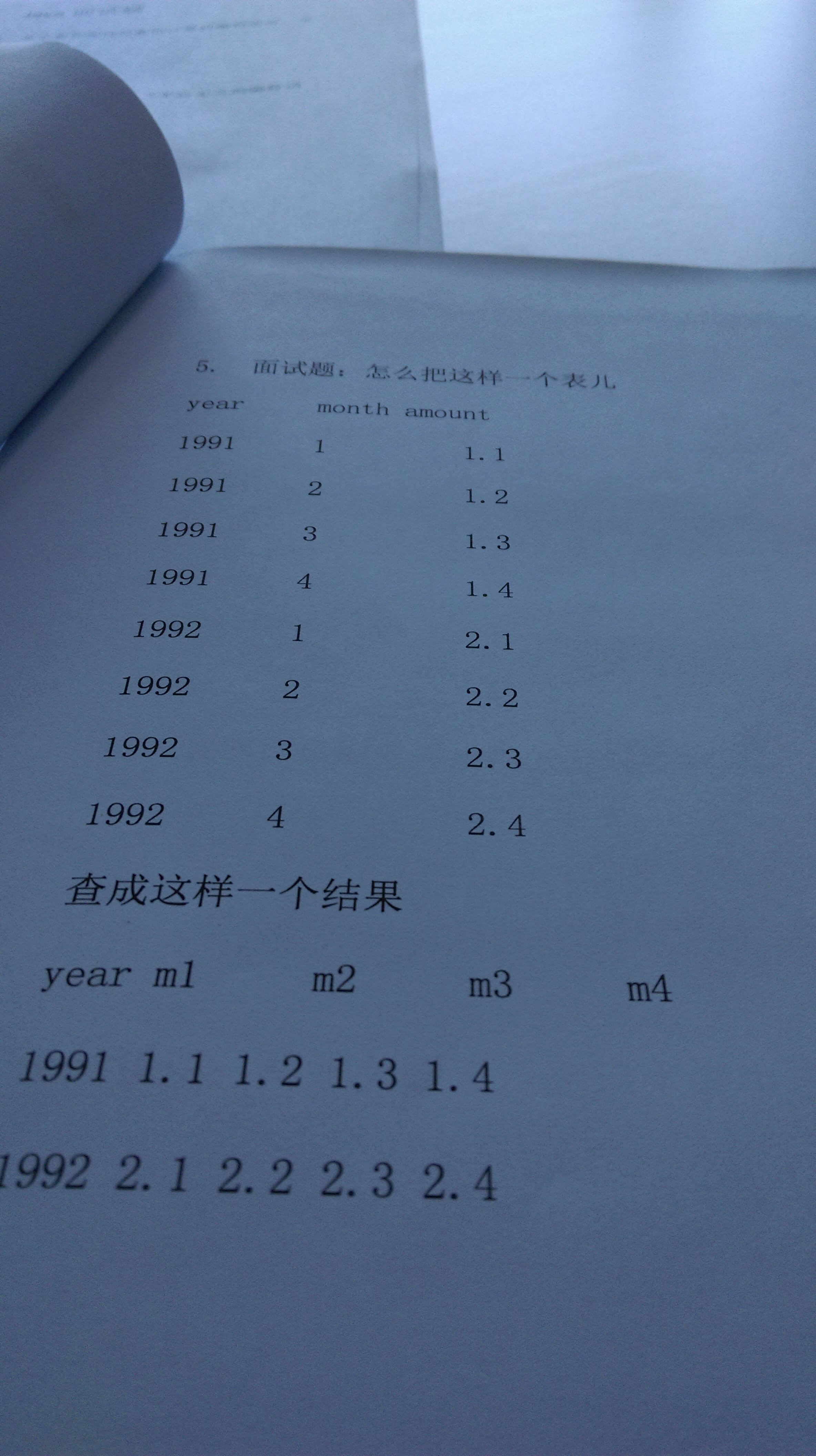mysql 这个查询怎么写3个回答

select year ,sum(case when month=1 then amount else 0 end) as m1
,sum(case when month=2 then amount else 0 end) as m2
,sum(case when month=3 then amount else 0 end) as m3
,sum(case when month=4 then amount else 0 end) as m4
from table
group by year

select g1.year,g1.account as m1,g2.account as m2,g3.account as m3,g4.account as m4 from group_test g1
left join group_test g2 on g1.year = g2.year and g2.month = 2
left join group_test g3 on g1.year = g3.year and g3.month = 3
left join group_test g4 on g1.year = g4.year and g4.month = 4
where g1.month = 1

select g1.year, g1.account as m1,g2.account as m2,g3.account as m3,g4.account as m4 from group_test g1
left join group_test g2 on g1.year = g2.year
left join group_test g3 on g1.year = g3.year
left join group_test g4 on g1.year = g4.year
group by year